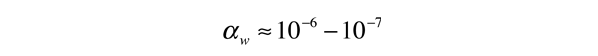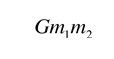# Coupling Constants for the Fundamental Forces

In attributing a relative strength to the four fundamental forces, it has proved useful to quote the strength in terms of a coupling constant. The coupling constant for each force is a dimensionless constant. The links below take you to some information about determining the value of the coupling constants.

 Coupling Constants Strong αs 1 Electromagnetic α 1/137 Weak αw 10-6 Gravity αg 10-39
Index

Fundamental force concepts

Reference
Rohlf
Ch. 1

 HyperPhysics***** Quantum Physics R Nave
Go Back

# Strong Force Coupling Constant

In obtaining a coupling constant for the strong interaction, say in comparison to the electromagnetic force, it must be recognized that they are very different in nature. The electromagnetic force is infinite in range and obeys the inverse square law, while the strong force involves the exchange of massive particles and it therefore has a very short range. It is clear that the strong force is much stronger simply from the fact that the nuclear size (strong force dominant) is about 10-15 m while the atom (electromagnetic force dominant) is about 10-10 m in size. From consideration of the "particle in a box" problem and from just the uncertainty principle, we know it takes greater energy to confine a particle to a smaller volume.

The body of data describing the strong force between nucleons is consistent with a strong force coupling constant of about 1:

αs ≈ 1

But the standard model sees the strong force as arising from the forces between the constituent quarks, which is called the color force. One of the discoveries about this force is that it dimishes inside the nucleons, so that the quarks are able to move freely within the hadrons. The implication for the strong force coupling constant is that it drops off at very small distances. This phenomenon is called "asymptotic freedom" because the quarks approach a state where they can move without resistance in the tiny volume of the hadron. Analysis of the coupling constant with quantum chromodynamics gives an expression for the diminishing coupling constant:Coupling constants for fundamental forces
Index

Fundamental force concepts

Reference
Rohlf
Ch. 1

 HyperPhysics***** Quantum Physics R Nave
Go Back

# Electromagnetic Force Coupling Constant

The strength of the electromagnetic force can be related to the force between two electrons given by Coulomb's law.

 The force isand the constanthas the units of energy times distance.

Another quantity with those dimensions which occurs naturally in the interaction of radiation with matter isA dimensionless constant which characterizes the electromagnetic force isThis coupling constant is also called the "fine structure constant" since it shows up in the description of the fine structure of atomic spectra. It appears naturally in the equations for many electromagnetic phenomena.

 Coupling constants for fundamental forces
 More detail on the fine structure constant
 Fine structure constant in Rutherford formula
Index

Fundamental force concepts

Reference
Rohlf
Ch. 1

 HyperPhysics***** Quantum Physics R Nave
Go Back

# Experimental Fine Structure Constant

As a dimensionless quantity α that appears in the equations of electromagnetic phenomena, the fine structure constant has almost the value 1/137. Until precise experiments demonstrated otherwise, it was often regarded as the reciprocal of the exact integer α = 1/137. This generated much discussion about how such a perfect integer could be a part of natural phenomena.

The fine structure constant can be expressed in terms of four fundamental physical constants, each of which has been measured with great precision, so that the value of the fine structure constant can be determined with a high level of precision.Substituting the experimental values for the four physical constants gives:Coupling constants for fundamental forces
 Fine structure constant in Rutherford formula
Index

Fundamental force concepts

References
Rohlf
Ch. 1

Fine structure constant, Wikipedia

 HyperPhysics***** Quantum Physics R Nave
Go Back

# Weak Force Coupling Constant

The weak interaction has such an incredibly short range that it's strength must be evaluated in a different way than the electromagnetic force. The fact that the both the strong force and the weak force initiate decays of particles gives a way to compare their strength. The lifetime of a particle is proportional to the inverse square of the coupling constant of the force which causes the decay. From the example of the decays of the delta and sigma baryons, the weak coupling constant can be related to the strong force coupling constant. This application givesSince the strong coupling constant has a value of about 1 in the energy range around 1 GeV, this suggests a value for the weak coupling constant in the rangeCoupling constants for fundamental forces
Index

Fundamental force concepts

Reference
Rohlf
Ch. 1

 HyperPhysics***** Quantum Physics R Nave
Go Back

# Gravity Force Coupling Constant

The strength of the gravitational force can be related to the force between two selected masses.

 The force isand the constanthas the units of energy times distance.

Since the masses and charges of basic particles like the electron and proton are independent of each other, the strength of the gravity force relative to the electromagnetic force depends upon which particles you choose for comparison. If two protons are chosen for the comparison, then.

Using the electromagnetic coupling constant of 1/137 then leads to a gravitational coupling constantIf the force between an electron and a proton is used, the comparison between gravitational and electric force is.
 Coupling constants for fundamental forces
Index

Fundamental force concepts

Reference
Rohlf
Ch. 1

 HyperPhysics***** Quantum Physics R Nave
Go Back